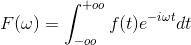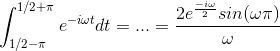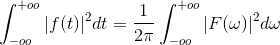# Find this integral

## Homework Statement

[PLAIN]http://www4d.wolframalpha.com/Calculate/MSP/MSP19019g3i9ie1bib5d9c00005gh8i4ce0eiab01f?MSPStoreType=image/gif&s=39&w=291&h=54 [Broken]

3. the attempt at a solution

I tried using the rectangular function and we know that

pT(t) is 1 for |t|<=T and 0 otherwise

hence for T = π

I get

pT(t) is 1 for |t|<=π and 0 otherwise

now also using the fourier transform I get that

sin(π*t)
_______ <----> π*pπ(-ω)
t

now I said that

sin(π*(1/2-t))
____________ <----------> π*pπ(ω-1/2)

1/2 - t

is this correct?

after using the Parseval theorem, I get for some reason π^2 and not 2π

[URL]http://latex.codecogs.com/gif.latex?x%20=%20\frac{1}{2%20\pi}\int_{-oo}^{+oo}%20\pi%20p_{\pi}%28\omega%29%20\pi%20p_{\pi}%28\omega%20-%201/2%29%20=%20\frac{pi}{2}%20\int_{-oo}^{+oo}%20p_{\pi}%28\omega%29%20p_{\pi}%28\omega%20-%201/2%29[/URL]

now since the set of all values in [-pi + 1/2, pi] are within [-pi, pi] and also from [pi,pi+1/2] all values in the integration will give 0

I will have to integrate 1 from -pi to pi and this way I get 2*pi which will give me π^2

Last edited by a moderator:

vela
Staff Emeritus
Homework Helper
now I said that

sin(π*(1/2-t))
____________ <----------> π*pπ(ω-1/2)

1/2 - t

is this correct?
No, this isn't correct. The function is even about t=1/2, so you have

$$\frac{1}{\sqrt{2\pi}}\int_{-\infty}^\infty \frac{\sin[\pi(\frac{1}{2}-t)]}{\frac{1}{2}-t} e^{i\omega t}\,dt = \frac{1}{\sqrt{2\pi}}\int_{-\infty}^\infty \frac{\sin[\pi(t-\frac{1}{2})]}{t-\frac{1}{2}} e^{i\omega t}\,dt = e^{i\omega/2}\left(\frac{1}{\sqrt{2\pi}}\int_{-\infty}^\infty \frac{\sin \pi t'}{t'} e^{i\omega t'}\,dt'\right)$$
(I don't know what convention you're using for the Fourier transform, so you may need to tweak the signs here and there.)

Note also that the integral the problem is asking you to evaluate is a convolution of $(\sin\pi t)/t$ with itself.

I'm using the following conventionI tried to find the fourier transform of the rectangular function for which it is known that

p1/2(x) is 1 for 1/2-π <= x <= 1/2 + π
and 0 otherwise

and I get this integralnow for parseval I use the followingBut after using the symmetric attribute of Fourier trasnform which says that

if f(t) <-> F(ω)
then

F(t) <-> 2π f(-ω)

and plug everything in, the left part of the equation in Parseval will not be what the exercise wants...

is it possible to transform it so that I will get the desired part?

edit: here is the integral again, because it seems like the picture disappeared
[PLAIN]http://img171.imageshack.us/img171/9761/msp12519g42h8iegc5gd850.gif [Broken]

Last edited by a moderator:
vela
Staff Emeritus
Homework Helper
I tried to find the fourier transform of the rectangular function for which it is known that

p1/2(x) is 1 for 1/2-π <= x <= 1/2 + π
and 0 otherwise
This doesn't match what you said before. Where is the pi coming from?
and plug everything in, the left part of the equation in Parseval will not be what the exercise wants...

is it possible to transform it so that I will get the desired part?
Don't use Parseval's theorem. Use the convolution theorem.

Note: Just put your LaTeX between [tеx] and [/tеx] tags to write equations. There's no need to link to an external site.

This doesn't match what you said before. Where is the pi coming from?

Don't use Parseval's theorem. Use the convolution theorem.

Note: Just put your LaTeX between [tеx] and [/tеx] tags to write equations. There's no need to link to an external site.

if I remember correct the convolution theorem says that for given two functions f,g, we have

$$\int_{-oo}^{+oo} f(t-x)g(x) dx$$

now should I find the fourier transform of these functions and then multiply them?

now I understand that we have an even function for $$sin(\pi (t-1/2))$$ which means that it will be the same as $$sin(\pi (1/2-t))$$

now by using the Fourier attribute of time shifting I have that

$$\frac{sin(\pi (t-1/2))}{t-1/2}$$ $\leftrightarrow$ $$\pi p_{\pi}(\omega) e^{\frac{-i \omega}{2}}$$

also we have

$$\frac{sin(\pi t)}{t}$$ $\leftrightarrow$ $$\pi p_{\pi}(\omega)$$

after this the result would be the multiplication of the two? I still can't find the result though, I think i've confused a little bit of everything :S

vela
Staff Emeritus
Homework Helper
You're heading in the right direction. Note the convolution of f(t) and g(t) yields another function:
$$(f*g)(t) = \int_{-\infty}^\infty f(t-x)g(x)\,dx$$
The integral you're asked to evaluate is
$$\int_{-\infty}^\infty \frac{\sin[\pi(\frac{1}{2}-x)]}{\frac{1}{2}-x}\frac{\sin \pi x}{x}\,dx$$
which is the convolution of $(\sin \pi t)/t$ with itself, evaluated at t=1/2. The idea is to use Fourier transforms to find the convolution and then set t=1/2 to get the final answer.

well

I found the fourier transform of the two functions in the integral, but then if I multiply I will get the fourier transform of the convolution of these two functions...

I'm a little bit confused, if I multiply

$$\pi p_{\pi}(\omega)$$ with $$\pi p_{\pi}(\omega) e^{\frac{-i \omega}{2}}$$

won't this be the fourier transform of the convolution? so can I say that the answer will be

$$\pi p_{\pi}(\omega)*\pi p_{\pi}(\omega) e^{\frac{-i \omega}{2}}$$

if I find the invert fourier transform of this multiplication I get the integral I'm trying to find

vela
Staff Emeritus
Homework Helper
You missed the key point of my previous post. Consider the convolution f*f with f(t)=$(\sin \pi t)/t$. How is the integral you're asked to find related to it? What is the Fourier transform of f*f?

You missed the key point of my previous post. Consider the convolution f*f with f(t)=$(\sin \pi t)/t$. How is the integral you're asked to find related to it? What is the Fourier transform of f*f?

we have a function

$$\frac{sin(\pi t)}{t}$$ and the convolution of this will be

$$\int_{-oo}^{+oo} \frac{sin(\pi(\frac{1}{2}-t))}{pi(\frac{1}{2}-t)} \frac{sin(\pi t)}{t} dt$$

I find the fourier transform of the functions

$$\frac{sin(\pi(\frac{1}{2}-t))}{pi(\frac{1}{2}-t)}$$

and

$$\frac{sin(\pi t)}{t}$$

and then I multiply them to find the fourier transform of f*f.

the fourier transform of f*f will be

$$\int_{-oo}^{+oo} \frac{sin(\pi(\frac{1}{2}-t))}{pi(\frac{1}{2}-t)} \frac{sin(\pi t)}{t} e^{(-i\omega t)} dt$$

hence

$$\int_{-oo}^{+oo} \frac{sin(\pi(\frac{1}{2}-t))}{pi(\frac{1}{2}-t)} \frac{sin(\pi t)}{t} e^{(-i\omega t)} dt =$$ $$\pi p_{\pi}(\omega)*\pi p_{\pi}(\omega) e^{\frac{-i \omega}{2}}$$

so the relation is this extra $$e^{(-i*\omega t)}$$ ?

I can't remove it though because if I put where t = 0 then the initial integral will change also

vela
Staff Emeritus
Homework Helper
we have a function

$$\frac{sin(\pi t)}{t}$$ and the convolution of this will be

$$\int_{-oo}^{+oo} \frac{sin(\pi(\frac{1}{2}-t))}{pi(\frac{1}{2}-t)} \frac{sin(\pi t)}{t} dt$$
Nope, that's not correct. The convolution of two functions yields another function. The integral above is a number. Try again.

you mean the convolution is not correct? I'm using the integration of convolution for sin(πt)/t

what I'm saying is that if I find the convolution, then by multiplying the two fourier transforms, I will get the fourier transform of the convolution

why is this not correct? Our professor has some notes where there is this attribute :(

vela
Staff Emeritus
Homework Helper
I'm saying you're being really sloppy with the math, and it's leading to errors and confusion. If
$$f(t)=\frac{\sin \pi t}{t}$$
the convolution f*f is
\begin{align*}
(f*f)(t) &= \int_{-\infty}^\infty f(t-x)f(x)\,dx \\
&= \int_{-\infty}^\infty \frac{\sin \pi(t-x)}{t-x}\frac{\sin \pi x}{x}\,dx
\end{align*}
That integral is not the same as
$$\int_{-\infty}^\infty \frac{\sin \pi(1/2-x)}{1/2-x}\frac{\sin \pi x}{x}\,dx$$
The convolution integral yields a function of t while the latter integral is equal to a number, namely $2\pi$. The two integrals are related, but they're not the same. How are they related?

You're also not applying the convolution theorem correctly either. Forget about the specifics of this problem for now. What does the theorem tell you is the Fourier transform of f*f?

yes I was wrong

so we have

\begin{align*}
(f*f)(t) &= \int_{-\infty}^\infty f(t-x)f(x)\,dx \\
&= \int_{-\infty}^\infty \frac{\sin \pi(t-x)}{t-x}\frac{\sin \pi x}{x}\,dx
\end{align*}

the function f is the same hence the fourier transform of the convolution will be

the multiplication of $$\pi p_{\pi}(\omega)$$ with itself

hence it will be $$(\pi)^{2} * (p_{\pi}(\omega))^{2}$$

I still can't figure out how the $$\omega$$ is related to everything